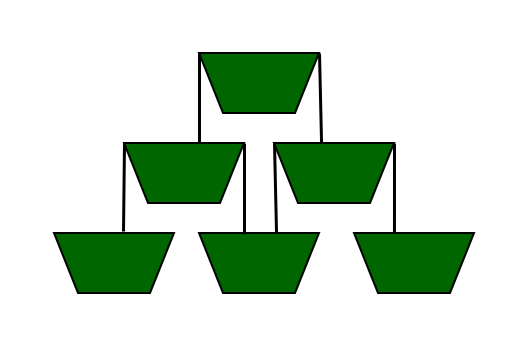# Number of containers that can be filled in the given time

Given a number N and a time X unit, the task is to find the number of containers that are filled completely in X unit if containers are arranged in pyramid fashion as shown below.
Note: Below example is the pyramid arrangement for N = 3, where N denotes the number of levels in the pyramid-shaped arrangement of containers such that level 1 has 1 container, level 2 has 2 containers and up to level N. The liquid is always poured in the topmost container at the 1st level. When the container of one level overflows from both its sides, the containers of the lower levels are filled. The amount of liquid poured in each second is equal to the volume of the container.Examples:

Input: N = 3, X = 5
Output: 4
After 1 second, the container at level 1 gets fully filled.
After 2 seconds, the 2 containers at level 2 are half-filled.
After 3 seconds, the 2 containers at level 2 are fully filled.
After 4 seconds, out of the 3 containers at level 3, the 2 containers at the ends are quarter-filled and the container at the center is half-filled.
After 5 seconds, out of the 3 containers at level 3, the 2 containers at the ends are half-filled and the container at the center is fully filled.

Input: N = 4, X = 8
Output: 6

## Recommended: Please try your approach on {IDE} first, before moving on to the solution.

Approach: This problem is solved using Greedy Approach. Below are the steps:

• After the container is getting filled, the liquid oveflows equally from both the sides of the container and fills the containers below it.
• Consider this pyramind of container as a matrix. So, cont[i][j] is the liquid in jth container of ith row.
• So, when overflow occurs, the liquid flows to cont[i+1][j] and cont[i+1][j+1].
• As the liquid will be poured for X seconds, so the total amount of liquid poured is X units.
• So the maximum value that can be poured into a container can be X units and minimum value that can be poured to completely fill it is 1 unit.

As the liquid is poured into the topmost container always, let the topmost container has a maximum value i.e. X units.
Steps for the algorithm are as follows:

1. Start the outer loop from 1 to N for n containers at each level. Inside this loop, start another loop for 1 to i, for each container of each row. Also declare a counter, count = 0, for counting the containers which are getting filled.
2. If value of cont[i][j] is greater than or equal to 1(it means it is filled) then, count is incremented by 1, and then in cont[i+1][j] & g[i+1][j+1] the liquid gets poured and their value is increased by value of (g[i][j]-1)/2 respectively, because the liquid is divided in half amount in both of them.
3. In this way continue the loop, and incrementing the countfor each filled container. When the loop ends our count will be the required answer.

Below is the implementation of the above approach:

 `// CPP program for the above problem ` `#include ` `using` `namespace` `std; ` ` `  `// matrix of containers ` `double` `cont; ` ` `  `// function to find the number ` `// of containers that will be ` `// filled in X seconds ` `void` `num_of_containers(``int` `n, ` `                       ``double` `x) ` `{ ` `    ``int` `count = 0; ` ` `  `    ``// container on top level ` `    ``cont = x; ` `    ``for` `(``int` `i = 1; i <= n; i++) { ` `        ``for` `(``int` `j = 1; j <= i; j++) { ` ` `  `            ``// if container gets filled ` `            ``if` `(cont[i][j] >= (``double``)1) { ` `                ``count++; ` ` `  `                ``// dividing the liquid ` `                ``// equally in two halves ` `                ``cont[i + 1][j] ` `                    ``+= (cont[i][j] ` `                        ``- (``double``)1) ` `                       ``/ (``double``)2; ` ` `  `                ``cont[i + 1][j + 1] ` `                    ``+= (cont[i][j] ` `                        ``- (``double``)1) ` `                       ``/ (``double``)2; ` `            ``} ` `        ``} ` `    ``} ` `    ``cout << count; ` `} ` ` `  `// driver code ` `int` `main() ` `{ ` `    ``int` `n = 3; ` `    ``double` `x = 5; ` `    ``num_of_containers(n, x); ` `    ``return` `0; ` `} `

Output:

```4
```

Time Complexity: O(N2)
Auxillary space Complexity: O(N2)

Attention reader! Don’t stop learning now. Get hold of all the important DSA concepts with the DSA Self Paced Course at a student-friendly price and become industry ready.

My Personal Notes arrow_drop_upCheck out this Author's contributed articles.

If you like GeeksforGeeks and would like to contribute, you can also write an article using contribute.geeksforgeeks.org or mail your article to contribute@geeksforgeeks.org. See your article appearing on the GeeksforGeeks main page and help other Geeks.

Please Improve this article if you find anything incorrect by clicking on the "Improve Article" button below.